• ### Browse All Lessons

##### Assign Lesson

Help Teaching subscribers can assign lessons to their students to review online!

 TweetThis lesson aligns with Next Generation Science Standards (NGSS) PS1.C

Introduction

Radioactive decay refers to the spontaneous disintegration of atomic nuclei. In other words, radioactivity is the emission of energy in the form of radiation. This phenomenon was first discovered in 1896 by the French physicist Henry Becquerel.  Due to nuclear instability, the elements or isotopes decompose spontaneously or decay by emitting radiations to attain a more stable nuclear configuration. Consequently, a significant amount of energy is released in the decay process.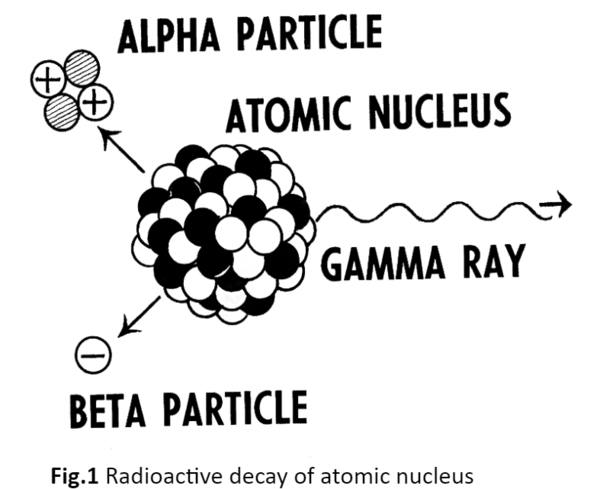Each radioactive element has a characteristic decay period or half-life. A half-life can be defined as the time required for one-half of the atomic nuclei of a radioactive sample to decay. For example, the radioisotope cobalt-60, which is significantly used in cancer therapy, has a half-life of 5.26 years. If an original sample contains 16 grams of cobalt-60, then after that interval of time, it would contain only 8 grams of cobalt-60 and emit half as much radiation. After another interval of 5.26 years, it would contain only 4 grams of cobalt-60.

The three most common types of radioactive decay are mentioned below. All of them involve emitting one or more particles.
• Alpha decay
• Beta decay
• Gamma decay

Alpha Decay
Alpha decay or -decay is a type of radioactive decay that involves the emission of an alpha particle by an atomic nucleus. The target nucleus transforms into a different atomic nucleus, with a mass number that is reduced by four and an atomic number that is reduced by two. The emitted alpha particle is identical to the nucleus of the helium-4 atom, which consists of two protons and two neutrons. Helium-4 is taken as a very stable alpha particle.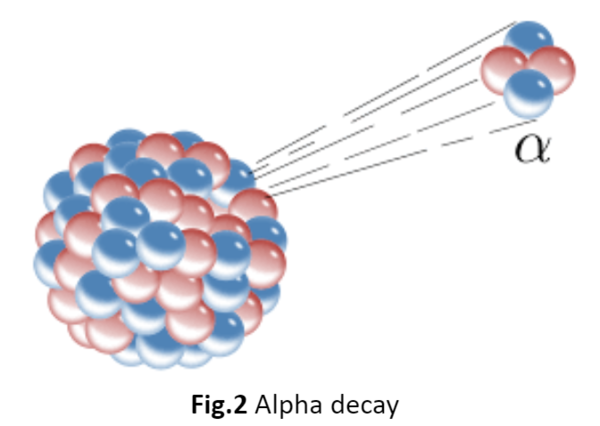The alpha particle or helium nucleus is preferentially emitted as compared to other particles due to the high binding energy of the alpha particle. This means that its mass is less than the sum of the masses of two protons and two neutrons. It increases the disintegration energy. The formula of alpha decay is given below:

$E = (m_i – m_f – m_p)c^2$

Where,
• $m_i$ is the initial mass of the nucleus.
• $m_f$ is the mass of the nucleus after particle emission.
• $m_p$mp is the mass of the emitted particle.
For instance, radium-226 decays into radon-222 and emits an alpha particle. The complete reaction is mentioned below: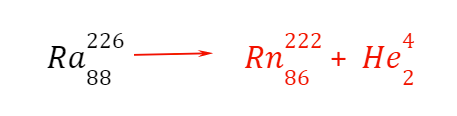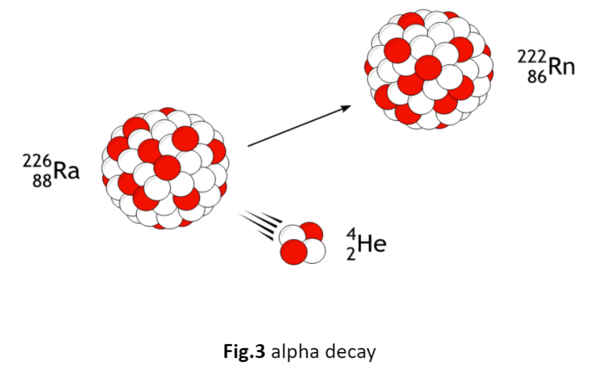Beta Decay
A beta particle is referred to as an electron, but it also can be a positron. -decay is a type of radioactive decay in which a fast, energetic electron or positron is emitted from an atomic nucleus. This process transforms the original nuclide into an isobar of that nuclide.

If the reaction involves electron emission, the nucleus sheds out neutrons one by one. The proton numbers also increase accordingly.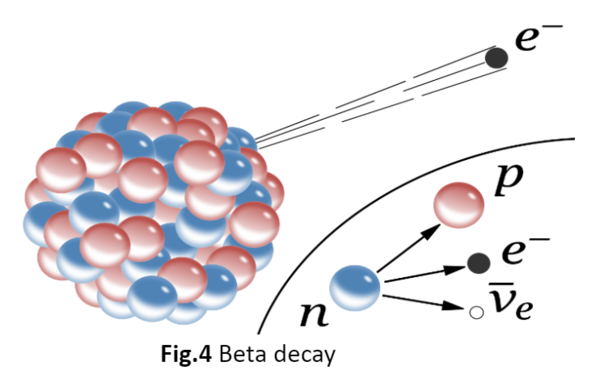For example, cesium-137 transformed into barium-137 with the emission of an electron.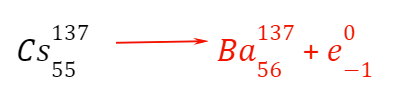Gamma Decay
Outside the nucleus, electrons are orbiting in fixed orbits, which indeed have some energy. When an electron jumps from a high energy state to a low energy state, there is an emission of a photon. Gamma emission occurs as the excited states transform into a lower energy state of the same nucleus. Gamma rays are photons without rest mass or charge.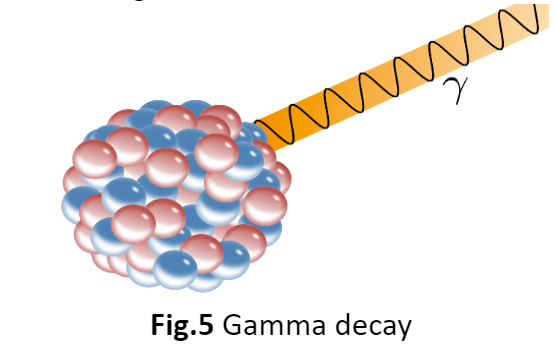Radioactive Decay LawAccording to the radioactive decay law, when a radioactive material undergoes a decay process, either α, β, or γ-decay, the number of nuclei undergoing the decay per unit of time is proportional to the total number of nuclei in the given sample material.

This law describes as “the probability per unit time that a nucleus will decay is a constant, independent of time”. The mathematical representation of this law is written below: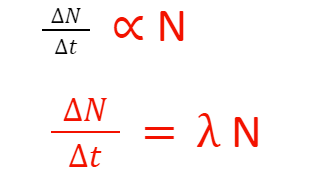N: the total number of nuclei in the sample
∆N: number of nuclei that undergoes the decay process
∆t: unit time: radioactive decay constant